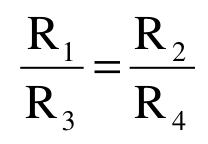# Wheatstone Bridge Circuit

The Wheatstone bridge is named after a British physicist Sir Charles Wheatstone. It is a type of electrical scale, which is mainly used to measure an unknown resistance. It can also be used in measuring strain, as temperature and control device and to amount other electrical values.

A Wheatstone bridge circuit arrangement is shown in the figure below.

FIGURE

It usually consists of four resistors with a voltage supply and two series connections in parallel. If the ratio R1 and R3 and the ratio of R2 and R4 are the same, then the voltage is measured as zero. And the bridge is said to be in balanced condition. By using the following equation, we can also determine the resistance value of unknown resistors.